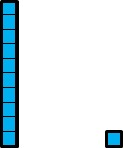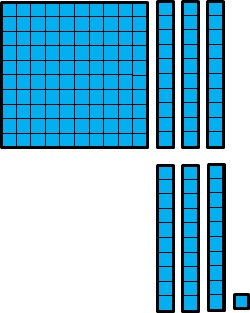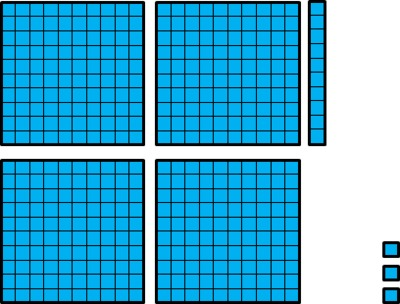# Drawing Pictures to Illustrate Decimal Comparisons

Alignments to Content Standards: 5.NBT.A.3.b

1. Which is greater, 0.01 or 0.001? Explain. Draw a picture to illustrate your explanation.

2. Which is greater, 0.03 or 0.007? Explain. Draw a picture to illustrate your explanation.

3. Which is greater, 0.025 or 0.052? Explain. Draw a picture to illustrate your explanation.

4. Which is greater, 0.13 or 0.031? Explain. Draw a picture to illustrate your explanation.

5. Which is greater, 0.203 or 0.21? Explain. Draw a picture to illustrate your explanation.

## IM Commentary

The purpose of this task is for students to compare decimal numbers using pictures or diagrams. Using such visual representations helps develop a deep understanding of the base-ten system and underscores that the relative place value of the digits can be more important than the value of the digits as numbers between 0 and 9. For students to be able to compare decimal numbers using these kinds of pictures, they should have familiarity with using base-ten blocks or bundled objects to represent decimal numbers in multiple ways. Tasks that can help develop this understanding are:

5.NBT Which number is it?

and

5.NBT Tenths and Hundredths.

## Solution

1. $0.01 \gt 0.001$ because 0.01 is 10 times bigger than 0.001.

In the picture, a small square represents 0.001 and ten of those small squares represents 0.01.2. $0.03 \gt 0.007$ because it takes 10 thousandths to make 1 hundredth, so 7 thousandths is smaller than 1 hundredth which is smaller than 3 hundredths.

In the picture, a small square represents 0.001 and ten of those small squares represents 0.01.3. $0.025 \lt 0.052$ because there are more hundredths in 0.052 than in 0.025, and hundredths are 10 times bigger than thousandths.

In the picture, a small square represents 0.001 and ten of those small squares represents 0.01. The pictures of the two numbers are stacked to make comparing them easier.4. $0.13 \gt 0.031$ because it takes 10 hundredths to make 1 tenth, so 0.031 is less than 1 tenth and 0.13 is greater than 1 tenth.

In the picture, a small square represents 0.001, ten of those small squares represents 0.01, and 100 of those small squares represents 0.1. The pictures of the two numbers are stacked to make comparing them easier.5. Lastly, $0.21 \gt 0.203$. They both have 2 tenths, but 0.21 has 1 hundredth, thereby making it greater than 0.203 which has 0 hundredths. In the picture, a small square represents 0.001, ten of those small squares represents 0.01, and 100 of those small squares represents 0.1.Note: pay close attention to the students' illustrations. They may make the 3 in 0.203 one base ten unit less than the 2. In this case, it will appear that 0.203 is greater than 0.21.